# Looking up and Combining text using TEXTJOIN and Array IF Statements.

in arrayformulainexcel •  2 years ago  (edited)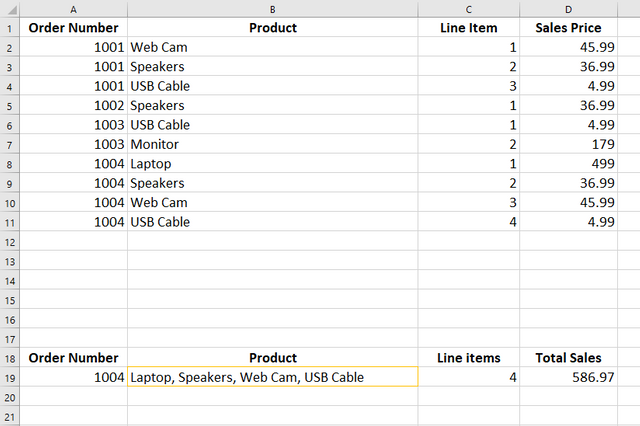Looking at the table of data below, how would you go about looking up the order number and pulling the name of all products sold on that order into the one cell as shown in cell B19 in the image below?Looks complex right? But not really with the use of TEXTJOIN and Array IF statements.

### What is an Array and how do you use array formula in Excel?

An array is a group of cells. An array formula is a formula where calculations are carried out on groups of cells.

Look at the GIF below. We wish to get the total price knowing there is 1 unit at 1.99 and 2 at 2.5 and 2 at 3.75 and 4 at 0.5.

We could multiply the price by the units for each item and then add all the prices together. We could also use the SUMPRODUCT function which does the same thing. But for this example, I want to show you an array.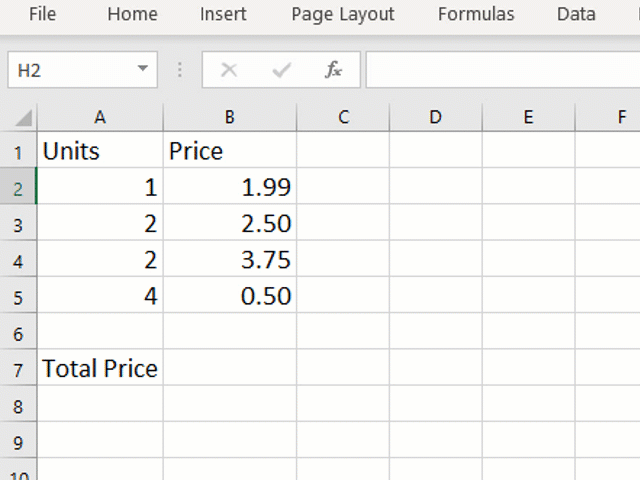We can also do this with a SUM array. Take a look at the following formula:

`=SUM(A2:A5*B2:B5)`
A2:A5 is an array. B2:B5 is also an array. This formula tells excel to multiply A2 by B2, then A3 by B3, then A4 by B4 then A5 by B5 and then sum the values together. But by pressing enter, this formula will not work.

When you are using array formula in excel you must press CTRL+SHIFT+ENTER to enter the formula. You will notice excel then places {} around the formula and the calculation can be carried out.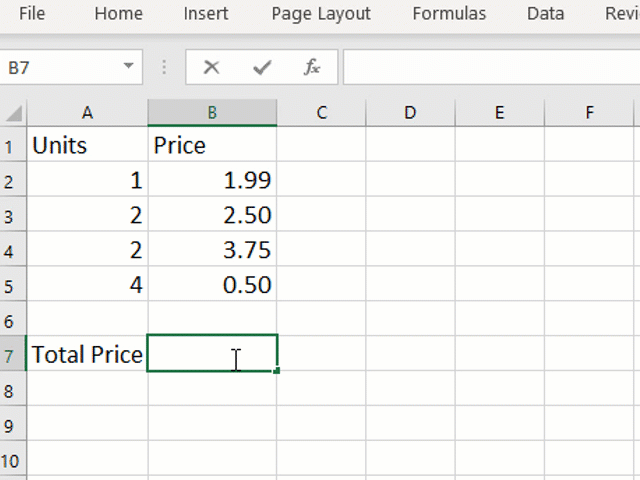### What is TEXTJOIN?

We covered TEXTJOIN in last weeks article and video, please do visit that post if you are any way confused.

TEXTJOIN will allow you join text from an array of cells into one cell and only specify the delimited once.

Its syntax is

`=TEXTJOIN(delimiter, Ignore empty, text1, text2….)`

### Combining TEXTJOIN with an array IF statement.

First we will take a look at the formula and then we will break it down so you can understand how it works.
`{=TEXTJOIN(", ",TRUE,IF(A2:A11=A19,B2:B11,""))}`
Okay now let’s explain it;

TEXTJOIN looks first for the delimiter, then choose between include or exclude empty cells. After this you then select the text that you want to join.

The first part of this formula is TEXTJOIN, where we want to separate each text item using a comma (“,” ) and we wish to ignore blanks (TRUE).

`=TEXTJOIN(", ",TRUE,`
The next part of TEXTJOIN is to add the cells that contain the text you want joined. TEXTJOIN lets you select both individual cells or an Array of cells. We can therefore use an array IF Statement to select the text we want.

An IF statement takes a logical test and allows you select the value to be shown if both true or false. In this case the logical test is an array logical test. If any of the cells between A2 and A11 are = A19 the we want the corresponding value from B2 to B11. If they are not a match, then we want blank (“”)

`IF(A2:A11=A19,B2:B11,""))`
This test will look and see of A2=B19 and if there is a match it will return the contents of cell B2. If no, it will return a blank. Next it will look at cell A3=A19 and if there is a match it will return the contents of cell B3. If no, t it will return a blank. This will continue down the array to cell A11.

The final part of this formula is telling Excel that it is an array. To do this we press CTRL + SHIFT + ENTER and you will see {} appear around the formula.

If we highlight IF(A2:A11=A19,B2:B11,"")) within the formula and press F9, we can see what’s going on here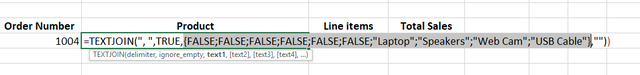The FALSE results represent each of the values in A2 to A11 that do not match A19, and we can see the product names where we do have a match.

In our IF statement we set blank (“”) to be the default where there is a FALSE. These blanks are then ignored by the TEXTJOIN function and so we are only returned with the products that match the Order number.

You can also view this with evaluate formula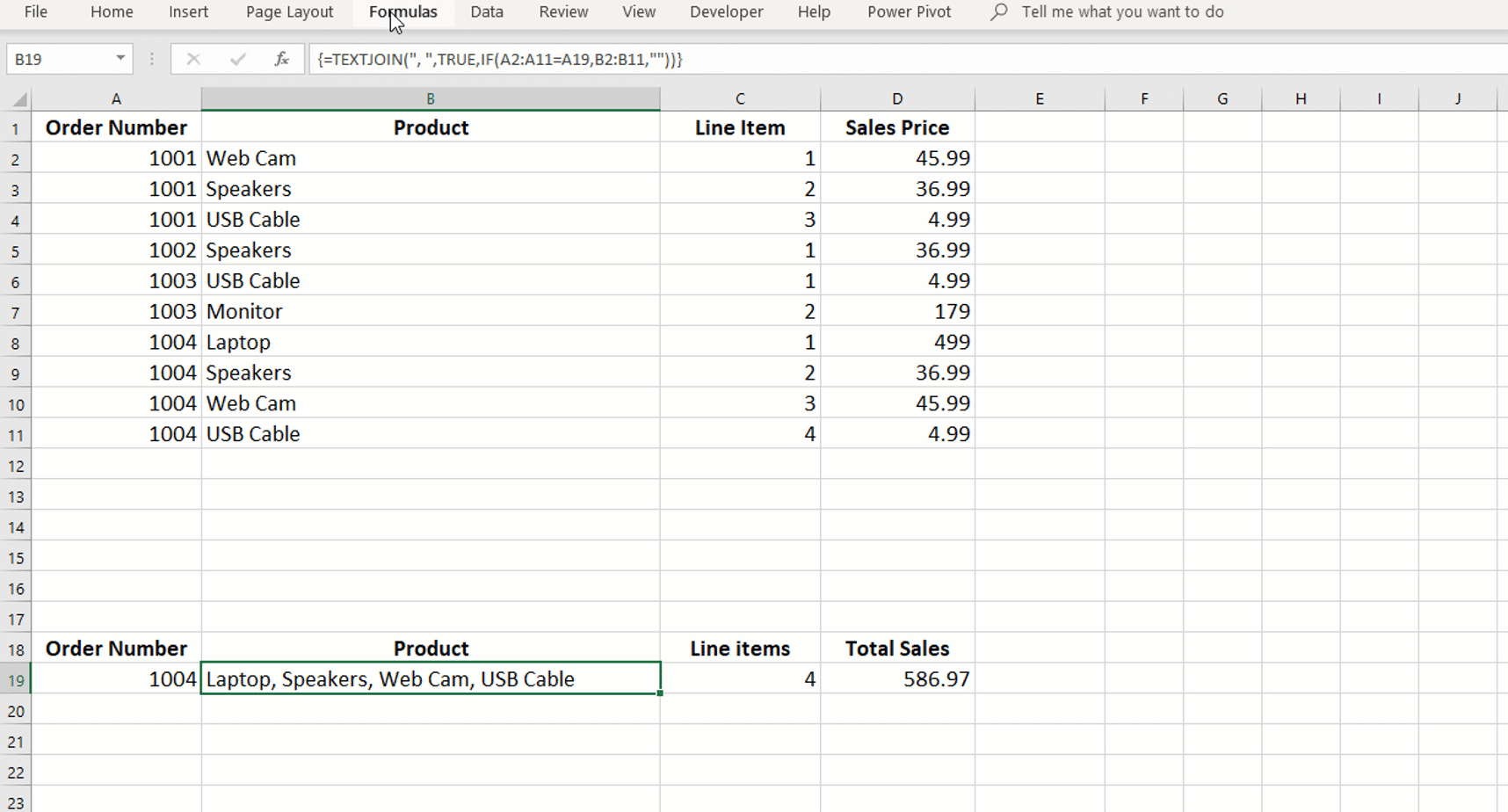#### What do you think would happen if we changed the TEXTJOIN formula to include the blank cells?

 Order Number Product Line Item Sales Price 1001 Web Cam 1 45.99 1001 Speakers 2 36.99 1001 USB Cable 3 4.99 1002 Speakers 1 36.99 1003 USB Cable 1 4.99 1003 Monitor 2 179 1004 Laptop 1 499 1004 Speakers 2 36.99 1004 Web Cam 3 45.99 1004 USB Cable 4 4.99

#### Sign up for my newsletter – Don’t worry, I wont spam. Just useful Excel and Power BI tips and tricks to your inbox

If you liked this posts – Looking up and Combining text using TEXTJOIN and Array IF Statements – Please do share. The share buttons are just below the comments!##### Now there is value in Learning with The Excel Club and our Learn and Earn STEEM activities.

We are the first Excel, PowerBI and DAX blog in the world where you can Earn while you Learn.

Cross posted from my blog with SteemPress : http://theexcelclub.com/looking-up-and-combining-text-using-textjoin-and-array-if-statements/

Sort Order:
·  2 years ago

great to see your excel postings here Paula.

I tried out this practice activity and was excepting to see ,,,, for the blanks and I was correct. This is what was returned to me , , , , , , 499, 36.99, 45.99, 4.99

·  2 years ago

Thank you very much Dernan. Yes you expectation was correct :-) the formula would return ,,,,, before the other items, however I see you have pulled back the sales price rather than the product names

·  2 years ago

Congratulations @theexcelclub! You have completed the following achievement on the Steem blockchain and have been rewarded with new badge(s) :You made more than 200 upvotes. Your next target is to reach 300 upvotes.

If you no longer want to receive notifications, reply to this comment with the word `STOP`